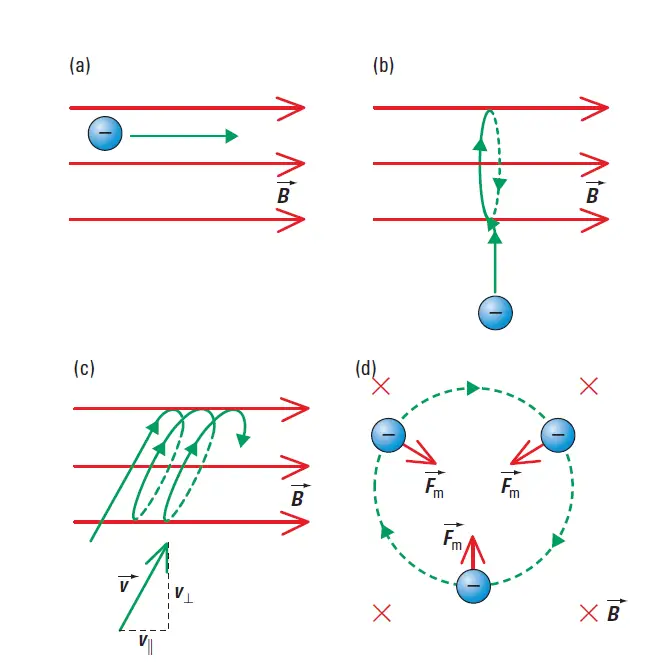# Path of a Charged Particle in a Uniform Magnetic Field

Last updated on August 24th, 2022 at 03:28 pm

Path of a Charged Particle in a Uniform Magnetic Field – The direction of the initial motion of a charged particle in an external magnetic field determines how the charged particle will deflect.

Figure 1 shows what can happen to a charged particle as it enters an external magnetic field:

## Path of a Charged Particle in a Uniform Magnetic Field

(a) If the initial motion of the charged particle is parallel to the external magnetic field, then there is no effect. When the charged particle’s velocity is parallel to the external magnetic field (B), the charged particle’s path is a straight line. (figure 1a)

(b) If the initial motion of the charged particle is perpendicular to the external magnetic field, the charge is deflected in a circular arc. The magnetic force acts as the centripetal force that causes the charge to follow a circular path. (figure 1b and figure 1d)

(c) If the initial motion of the charged particle is at an angle to an external magnetic field, the charge deflects in a circular motion that will form a helical path. (figure 1c)Path of a Charged Particle in a Uniform Magnetic Field (a) When the charged particle’s velocity is parallel to the external magnetic field (B), the charged particle’s path is a straight line. (b) The charged particle’s motion is perpendicular to the magnetic field, so the particle is deflected in a circular arc. (c) The charged particle’s motion is at an angle to the magnetic field, so the particle follows a helical path. (d) This side view from the left shows the magnetic force acting as the centripetal force that causes the charge to follow a circular path.

Oppositely charged particles deflect in opposite directions in a magnetic field.

If the magnitude of the external magnetic field is large enough, the field can cause circular motion that remains contained in the magnetic field. In this circular motion, the centripetal force is the magnetic force. (figure 1d)